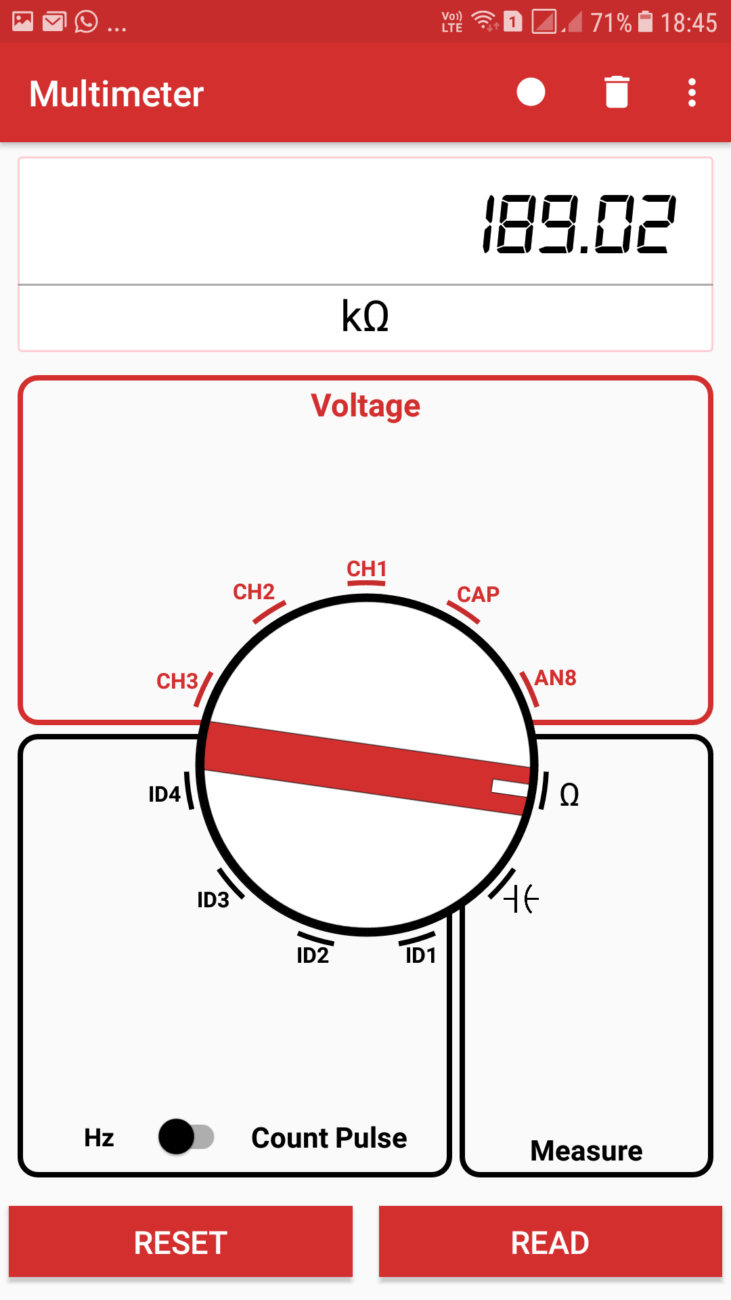# Measuring Current in PSLab Android App

The Pocket Science Lab Android app has got various functionality such as voltmeter, resistance measurement, capacitance measurement, frequency measurement as well as Count pulse measurement , one of the missing functionality among these is the Ammeter, currently in PSLab there is no direct way of measuring current. In this blog I will be discussing an indirect method of measuring current in PSLab.

## Basics of measuring current

Generally in all multimeters , current is measured using  an Ammeter which uses the property of galvanometer to measure to measure current in PSLab. But as the PSLab doesn’t has any such embedded Galvanometer we cannot have a seperate Ammeter in it, but there is another method to measure the current, which is using the famous OHm’s law i.e V/I = R

In PSLab we can measure the voltage across any elements, plus we can also measure  the resistance of any circuit element in PSLab, the theory used for measuring current is stated as follows.

1. We connect the current-source to any known resistant(or any resistance) and then measure the voltage across the resistor.
2. Finally using ohm’s law the current will be voltage / resistance.

## Step-by-step guide on measuring the current

Here is the step by step guide on how to measure the current in PSLab:-

1. Take any arbitrary resistor and measure it’s resistance. To measure the resistance follow these steps :-
1. Take a resistance and connect it to any two pins of breadboard
2. Connect one end to the sen pin and the other end to the GND pin of PSLab device.
3. Now go to the Android app and select multimeter instrument.
4. Now in the multimeter instrument set the knob to resistance, in the measure section
5. Now click on the read button and then it will show the resistance of the resistor
2. Now that you have measured the resistance of the resistor, now connect the current source across the resistors.
3. Now finally measure the voltage across the resistor. To measure the voltage follow these steps.
1. Take two male to male wires and connect it’s two individual ends to the two ends of the resistor.
2. Now connect one of the other two ends of the wire to the any one of the CH1, CH2 or CH3 and the other end to GND respectively.
3. Now go to the Android app and select multimeter instrument.
4. Now in the multimeter instrument set the knob to the channel which you have selected i.e CH1, CH2 or CH3 , in the voltage section.
5. Now click on the read button and then it will show the voltage across the resistor.
4. Now using the using ohm’s law the current will be voltage / resistance.

Thus using these steps one can find the current in PSLab Android App.

## Images for the ExperimentFigure (1): Showing resistance measurementFigure(2) Showing voltage measurement

## Resources

1. Using-a-Multimeter, dengarden.com:
https://dengarden.com/home-improvement/Using-a-Multimeter
2. Wikipedia, Ohm’s Law:
https://en.wikipedia.org/wiki/Ohm%27s_law